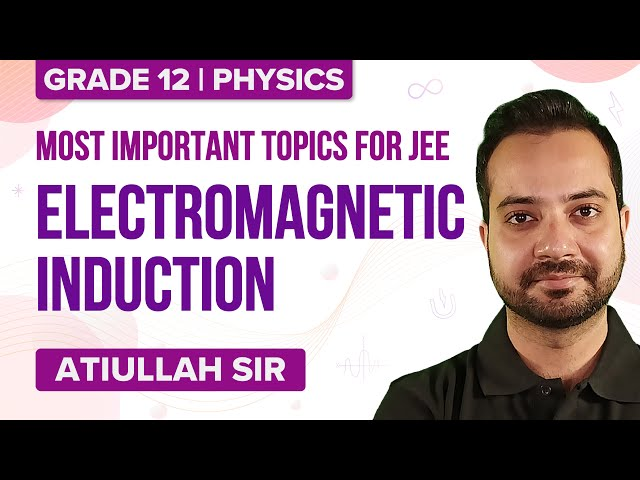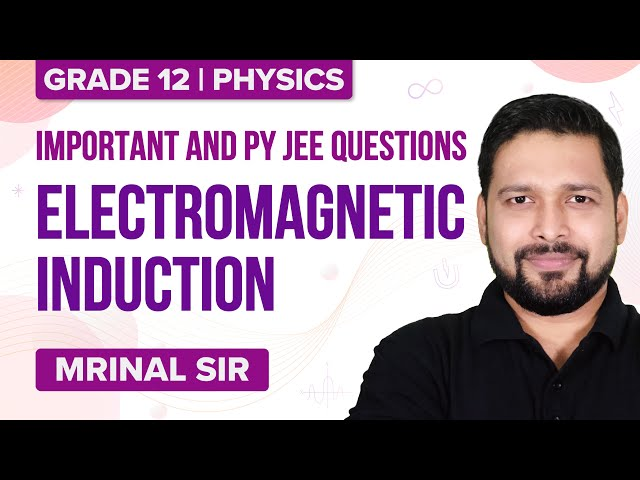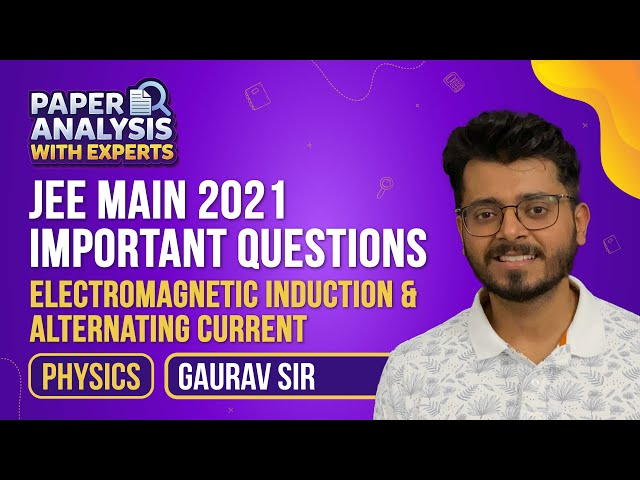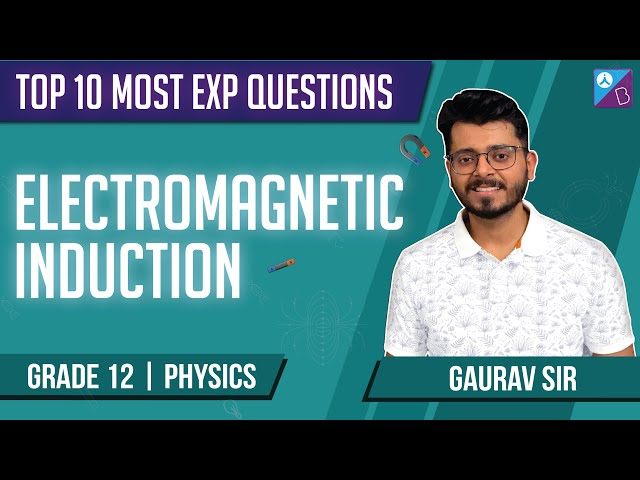Checkout JEE MAINS 2022 Question Paper Analysis : Checkout JEE MAINS 2022 Question Paper Analysis :

# What is Electromagnetic Induction?

Electromagnetic induction is a phenomenon in which the relative motion between a conductor and a magnetic field produces a potential difference (voltage) across the conductor.

### Magnetic Flux

Magnetic flux is the number of magnetic field lines passing through a given closed surface. It gives the measurement of the total magnetic field that passes through a given surface area. The area under consideration can be of any size and under any orientation with respect to the direction of the magnetic field.

The Magnetic Flux in a magnetic field

$$\begin{array}{l}\mathbf{\overrightarrow{B}}\end{array}$$
through an area
$$\begin{array}{l}\mathbf{\overrightarrow{dA}}\end{array}$$
is given by:

Magnetic Flux

$$\begin{array}{l}\mathbf{\Phi_{B}\;=\;\int \;\overrightarrow{B}\;.\;\overrightarrow{dA}}\end{array}$$

SI unit = Weber (Wb.) or Tesla

$$\begin{array}{l}\mathbf{m^{2}}\end{array}$$
.
$$\begin{array}{l}\mathbf{\left [ 1\; Tesla\;(T)\;=\;\frac{Weber\;(wb)}{m^{2}} \right ]}\end{array}$$

The Dimensions of Magnetic Flux is given by

$$\begin{array}{l}\mathbf{ M\; L^{2}\; T^{-2}\; A^{-1}}\end{array}$$

The Magnetic Flux associated with an elemental area

$$\begin{array}{l}\mathbf{\overrightarrow{dA}}\end{array}$$
in a magnetic field
$$\begin{array}{l}\mathbf{\overrightarrow{B}}\end{array}$$
is given by

$$\begin{array}{l}\mathbf{d\;\Phi_{B}\;=\; \overrightarrow{B}\;.\;\overrightarrow{dA}\;=\;B\;dA\;cos \; \theta }\end{array}$$
[Where,
$$\begin{array}{l}\mathbf{ \theta }\end{array}$$
= Angle between B and normal to the surface]

## Important Cases

Case 1: When

$$\begin{array}{l}\mathbf{ \theta \;=\;0}\end{array}$$

If the area

$$\begin{array}{l}\mathbf{\overrightarrow{dA}}\end{array}$$
is perpendicular to the direction of the magnetic field
$$\begin{array}{l}\mathbf{\overrightarrow{B}}\end{array}$$
then the magnetic flux associated with the area will me maximum i.e.
$$\begin{array}{l}\mathbf{d\;\Phi_{B}\;=\;B\;dA}\end{array}$$

Case 2: When

$$\begin{array}{l}\mathbf{ \theta \;=\;90}\end{array}$$

If the area

$$\begin{array}{l}\mathbf{\overrightarrow{dA}}\end{array}$$
lies along the direction of the magnetic field
$$\begin{array}{l}\mathbf{\overrightarrow{B}}\end{array}$$
then the magnetic flux associated with the area will be zero. i.e.
$$\begin{array}{l}\mathbf{d\;\Phi_{B}\;=\;0}\end{array}$$

### Gauss Law Of Magnetism

The total magnetic flux associated with the closed surface is always zero i.e.

$$\begin{array}{l}\mathbf{\oint \;\overrightarrow{B}\;.\;\overrightarrow{dA}\;=\;0}\end{array}$$

### Faraday’s Laws Of Electromagnetic Induction

Faraday’s First Law: The Faraday’s First law of Electromagnetic Induction states that the EMF is induced in the conductor whenever the magnetic flux linked with the coil changes or the moving conductor cuts the Magnetic flux. This phenomenon is known as Electromagnetic Induction and this induced EMF causes a flow of current in the closed circuit known as the Induced current.

Faraday’s Second Law: It states that the EMF induced in the coil is equal to the rate of change of magnetic flux linked with the coil.

The magnitude of EMF induced (e) is given by:

$$\begin{array}{l}\mathbf{e\;=\;-\;\frac{d\;\Phi }{dt}\;=\;\frac{\Phi _{2}\;-\;\Phi _{1}}{dt}}\end{array}$$

Where,

$$\begin{array}{l}\mathbf{\Phi _{1}\;\;-\;\;\Phi _{2}}\end{array}$$
= change in the magnetic flux linked with the coil in time (t). The negative sign indicates that the EMF induced opposes the changes in the magnetic flux (Lenz’s Law).

In the SI system, the induced EMF (e) is measured in volt (V) and Magnetic flux

$$\begin{array}{l}\mathbf{(\Phi)}\end{array}$$
is measured in weber (Wb).

Induced Current: The current is induced in the coil only if the circuit is closed. However, during the electromagnetic induction, an EMF is always induced whether the circuit is open or closed. Therefore, if R is the total resistance and E is the induced emf then,

Induced Current in a closed circuit

$$\begin{array}{l}\mathbf{i\;=\;\frac{e}{R}}\end{array}$$

If there are total N number of turns in the coil then,

$$\begin{array}{l}\mathbf{i\;=\;\frac{N}{R}\; \times \;\frac{d\Phi }{dt}}\end{array}$$

Induced Charge: The total charge flowing through the circuit as a result of induced current due to the electromagnetic induction is given by

Since

$$\begin{array}{l}\mathbf{i\;=\;\frac{e}{R}\;=\;\frac{1}{R}\;\frac{d\Phi }{dt}}\end{array}$$
and
$$\begin{array}{l}\mathbf{i\;=\;\frac{dq }{dt}}\end{array}$$

Therefore,

$$\begin{array}{l}\mathbf{dq\;=\;\frac{1}{R}\;\;d\Phi }\end{array}$$

Now,

$$\begin{array}{l}\mathbf{q\;=\;\int_{0}^{q}\;\;dq}\end{array}$$

i.e.

$$\begin{array}{l}\mathbf{q\;=\;\int_{\Phi _{1}}^{\Phi _{2}}\;\;\frac{1}{R}\;d\Phi \;=\;\frac{\Phi _{2}\;-\;\Phi _{1}}{R}}\end{array}$$

If there are N number of turns in the coil then,

$$\begin{array}{l}\mathbf{q\;=\;\frac{N\;\left (\Phi _{2}\;-\;\Phi _{1} \right )}{R}}\end{array}$$

### EMF Induced In The Moving Conductor

If a straight conductor of length l is moving perpendicular to a uniform magnetic field (B) with velocity (v) then,

Induced EMF:

$$\begin{array}{l}\mathbf{e\;=\;B\;l\;v}\end{array}$$

Induced Current:

$$\begin{array}{l}\mathbf{i\;=\;\frac{e}{R}\;=\;\frac{\;B\;l\;v}{R}}\end{array}$$
[R = Resistance of the conductor]

Self Inductance (L): The magnetic flux

$$\begin{array}{l}\mathbf{(\Phi )}\end{array}$$
linked with the coil through which the current (I) is flowing is given by

$$\begin{array}{l}\mathbf{\Phi \;=\;L\;I}\end{array}$$

The instantaneous induced EMF produced in the coil is given by

$$\begin{array}{l}\mathbf{e\;=\;\;L\;\frac{dI}{dt}}\end{array}$$

Where L = Coefficient of Self inductance

The Self Inductance of a Solenoid is given by

$$\begin{array}{l}\mathbf{L\;=\;\frac{\mu _{0}\;N^{2}\;A}{l}}\end{array}$$

Or,

$$\begin{array}{l}\mathbf{L\;=\;\frac{\mu _{0}\;n^{2}\;l^{2}\;A}{l}\;=\;\mu _{0}\;n^{2}\;l\;A}\end{array}$$

Where,

μ0 = permeability of a free space

n = number of turns per unit length, l = length of the coil

N = total number of turns [N = n l]

A = cross-sectional area of the coil

Mutual Inductance (M): The magnetic flux

$$\begin{array}{l}\mathbf{(\Phi )}\end{array}$$
linked with the secondary coil when the current (I) is flowing through the primary coil is given by

$$\begin{array}{l}\mathbf{\Phi \;=\;M\;I}\end{array}$$

The instantaneous induced EMF produced in the coil is given by

$$\begin{array}{l}\mathbf{e\;=\;\;M\;\frac{dI}{dt}}\end{array}$$

Where M = Coefficient of Mutual inductance

The Mutual Inductance between two coils is given by

$$\begin{array}{l}\mathbf{M\;=\;\mu _{r}\;\mu _{0}\;\frac{N_{1}\;N_{2}\;A}{l}}\end{array}$$

Where,

μr = relative permeability of the material

μ0 = permeability of a free space

A = Cross-sectional area of the coil

l = Length of the Primary and Secondary coil respectively

N1 and N2 = Total number of turns in the Primary and Secondary coil respectively

## Electromagnetic Induction – Important Topics## Electromagnetic Induction – Important Questions### Electromagnetic Induction Important JEE Main Questions### Electromagnetic Induction – Top 10 Important and Expected JEE QuestionsTest your knowledge on Electromagnetic Induction For Iit Jee